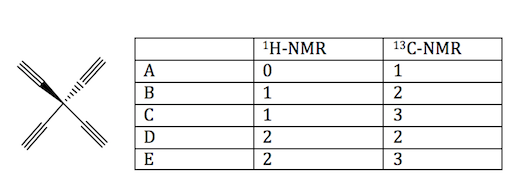Problem: How many signals would you expect for the 1H-NMR and 13C-NRM spectra of the following compounds respectively?

FREE Expert Solution
96% (410 ratings)
Problem Details

How many signals would you expect for the 1H-NMR and 13C-NRM spectra of the following compounds respectively?What scientific concept do you need to know in order to solve this problem?

Our tutors have indicated that to solve this problem you will need to apply the 1H NMR: Number of Signals concept. You can view video lessons to learn 1H NMR: Number of Signals Or if you need more 1H NMR: Number of Signals practice, you can also practice 1H NMR: Number of Signals practice problems .

What is the difficulty of this problem?

Our tutors rated the difficulty of How many signals would you expect for the 1H-NMR and 13C-NRM... as low difficulty.

How long does this problem take to solve?

Our expert Organic tutor, Nicolle took 2 minutes to solve this problem. You can follow their steps in the video explanation above.

What professor is this problem relevant for?

Based on our data, we think this problem is relevant for Professor Closser's class at CSU.